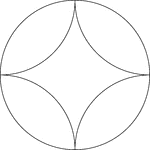### Arcs Inscribed In A Circle

A design created by inscribing 4 congruent tangent arcs in a circle.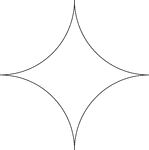### Reflected Arcs Of A Circle

A design created by dividing a circle into 4 equal arcs and reflecting each arc toward the center of…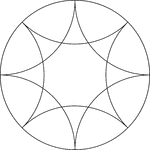### Reflected Arcs Of 2 Circles In A Circle

A design created by dividing a circle into 4 equal arcs and creating a reflection of each arc toward…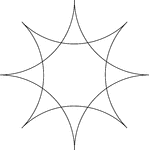### Reflected Arcs Of 2 Circles

A design created by dividing a circle into 4 equal arcs and reflecting each arc toward the center of…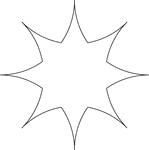### Reflected Arcs Of 2 Circles

A design created by dividing a circle into 4 equal arcs and reflecting each arc toward the center of…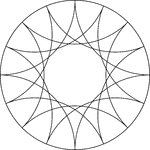### Reflected Arcs Of 4 Circles In A Circle

A design created by dividing a circle into 4 equal arcs and creating a reflection of each arc toward…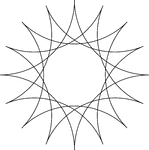### Reflected Arcs Of 4 Circles In A Circle

A design created by dividing a circle into 4 equal arcs and creating a reflection of each arc toward…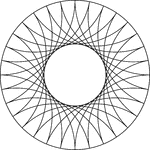### Reflected Arcs Of 8 Circles In A Circle

A design created by dividing a circle into 4 equal arcs and creating a reflection of each arc toward…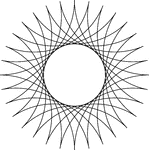### Reflected Arcs Of 8 Circles

A design created by dividing a circle into 4 equal arcs and creating a reflection of each arc toward…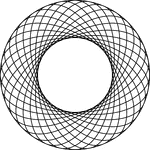### 16 Rotated Concentric Ellipses

Illustration of 16 concentric congruent ellipses that are rotated about the center at equal intervals…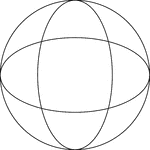### 2 Rotated Concentric Ellipses

Illustration of 2 concentric congruent ellipses that are rotated about the center at 90°. The ellipses…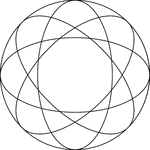### 4 Rotated Concentric Ellipses

Illustration of 4 concentric congruent ellipses that are rotated about the center at equal intervals…### 8 Rotated Concentric Ellipses

Illustration of 8 concentric congruent ellipses that are rotated about the center at equal intervals…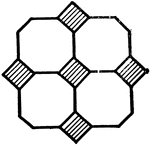### Design Consisting of Octagons and Squares

Illustration of a figure that is made up of 4 octagons with 5 squares (diamonds).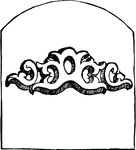### Scroll

A convolved or spiral ornament, variously introduced.